The VIRGO project: Cosmological N-body Simulations

We have carried out the following 3 sets of intermediate-size (not quite appropriate, as they are still large enough!) N-body simulations:
The page here gives you some information of our work.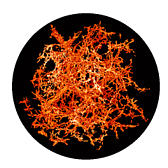Pictures and movies here.

VIRGO simulations

 Simulation parameters

Shown in the left panels are projected mass distibutions in a sliced region in the simulation box. Low-density regions which look like "voids" are dark, while red (and yellow) parts are high-density regions. The pictures were created using an adaptive smoothing scheme which assigns to each particle an individual smoothing length. Thus, high density regions are not smoothed at all whereas low density regions are smoothed. (Pictures created by Joerg Colberg)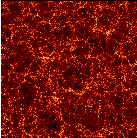Boxsize 239.5 Mpc/h 2563 particles OMEGA = 1.0 LAMBDA = 0.0 H0 = 50 km/(Mpc sec) Sigma8 = 0.51 Mass per particle 22.7 1010M_sun/h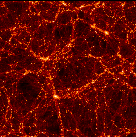Boxsize 239.5 Mpc/h 2563 particles OMEGA = 0.3 LAMBDA = 0.7 H0 = 70 km/(Mpc sec) Sigma8 = 0.9 Mass per particle 6.86 1010M_sun/h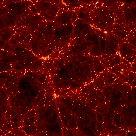Boxsize 239.5 Mpc/h 2563 particles OMEGA = 0.3 LAMBDA = 0.0 H0 = 70 km/(Mpc sec) Sigma8 = 0.85 Mass per particle 6.86 1010M_sun/h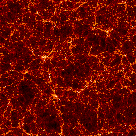Boxsize 239.5 Mpc/h 2563 particles OMEGA = 1.0 LAMBDA = 0.0 H0 = 50 km/(Mpc sec) Sigma8 = 0.51 Mass per particle 22.7 1010M_sun/h

 Suitable references
(Virgo-simulation selected publications):
• Evolution of Structure in Cold Dark Matter Universes, A. Jenkins, C.S.Frenk, F.R.Pearce, P.A.Thomas, J.M.Colberg, S.D.M.White, H.M.P Couchman, J.A.Peacock, G.Efstathiou, and A.H.Nelson (The Virgo Consortium), 1998, Astrophysical Journal,499,20-40.

• The structure of galaxy clusters in various cosmologies, P.A.Thomas,J.M.Colberg,H.M.P Couchman, G.Efstathiou, C.S.Frenk, A. Jenkins, A.H.Nelson, R.M.Hutchinhgs, J.A.Peacock, F.R.Pearce, S.D.M.White, 1998,MNRAS,296,1061

Back to
MPA/ NumCos